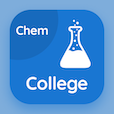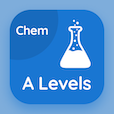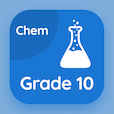Online College Courses

College Chemistry MCQs

College Chemistry MCQ - Topics

Learn Moles Multiple Choice Questions (MCQ Quiz), Moles quiz answers PDF to learn college chemistry course for college chemistry online classes. Basic Chemistry Multiple Choice Questions and Answers (MCQs), Moles quiz questions for colleges that offer online degrees. Moles Book PDF: spectrometer, empirical formula, relative abundance test prep for best GRE prep courses online.

"Moleis defined as the mass of an element divided by" MCQ PDF: moles App APK with molar mass, atomic number, atomic mass, and empirical formula choices for colleges that offer online degrees. Study moles quiz questions for merit scholarship test and certificate programs for online schools that offer certificate programs.

## MCQs on Moles Quiz

MCQ: Moleis defined as the mass of an element divided by

Molar mass
atomic number
atomic mass
empirical formula

MCQ: The molecules expressed by formula unit mass are

atoms
neutral gases
covalent
ionic

MCQ: The mass of one molecule of sulphuric acid is

98
95
97
92

MCQ: The gram atomic mass of usually occurring uranium is

239
238
235
236

MCQ: The masses of atoms or molecules expressed in grams is known as

Molar mass
empirical mass
molecular formula mass
none of above

### More Quizzes on College Chemistry Book